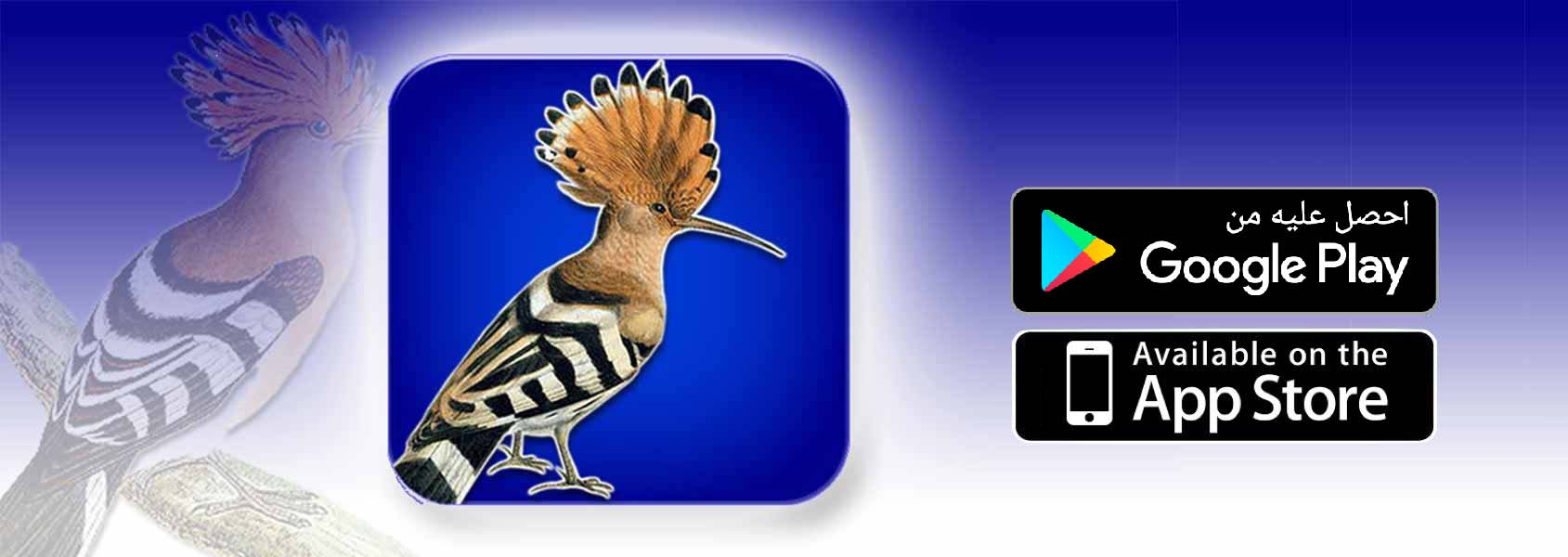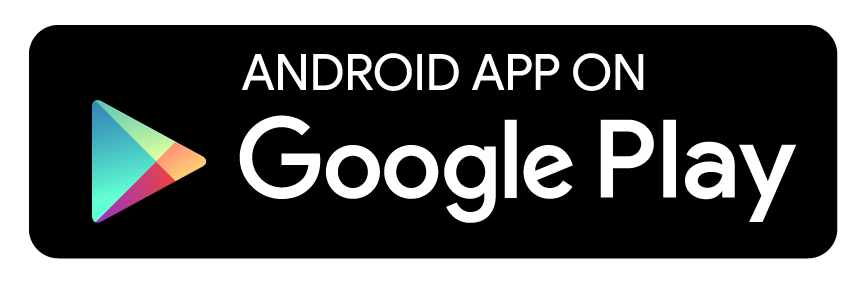تعلم اللهجه الاماراتيه في دقايق الشبريه = السرير كبت = خزانه الملابس مسيد = مسجد هيه = نعم ( إيه ) بسير = بروح. بوش = مجموعه الجمال ( النوق ) غرشه = زجاجه موتر = سيارة كشخه = زين او تعبر عن اعجابك بشئ الدوبي= اللي يكوي الملابس سامان= أغراض شرات = مثل وين سايرين=وين رايحين اخطف عليك = امر عليك ارمس= تكلم ربيع = صديق ما تيوز = ما تترك خلاف = بعدين أزغر فلان: ناده المزغوده = المخنوقه

مسجات متنوعة

January 22nd 2015, 10:55 am